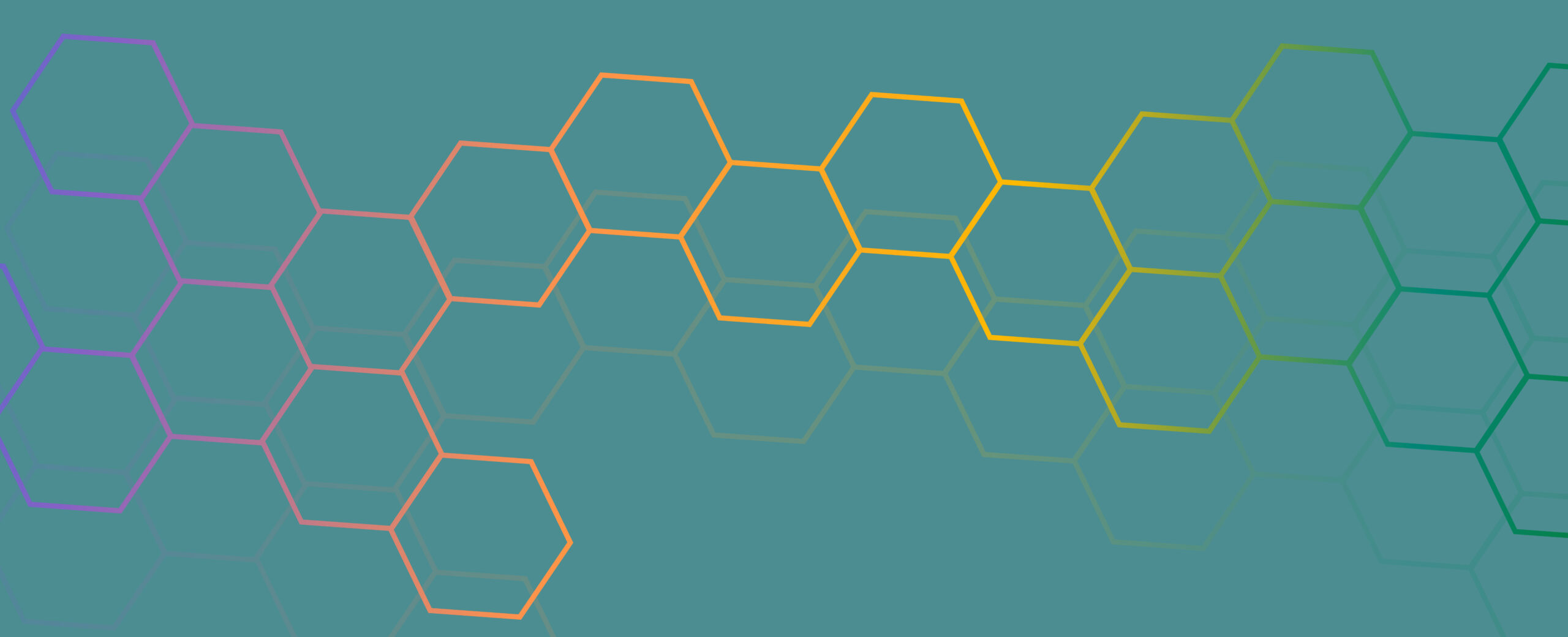# Introducing CliffordLayers: Neural Network layers inspired by Clifford / Geometric Algebras.

We are open sourcing CliffordLayers, a repo for building neural network layers inspired by Clifford / Geometric Algebras. This repo contains the source code of our ICLR 2023 paper Clifford Neural Layers for PDE Modeling and will be populated by the new geometric layers introduced in paper Geometric Clifford Algebra Networks.

Partial differential equations (PDEs) see widespread use in sciences and engineering to describe simulation of physical processes as scalar and vector fields interacting and coevolving over time.

Below, we show an example of a scalar field – the pressure field – on the left, and a vector field – the wind velocity field – on the right. Those fields interact with each other. When pressure gradients occur, i.e., when the pressure field changes, the velocity field changes too. Consequently, net air movement from high to low pressure regions arises, which in turn affects the pressure field. Those two fields are intrinsically coupled, and it intuitively makes sense to treat them as one object instead of three different channel dimensions.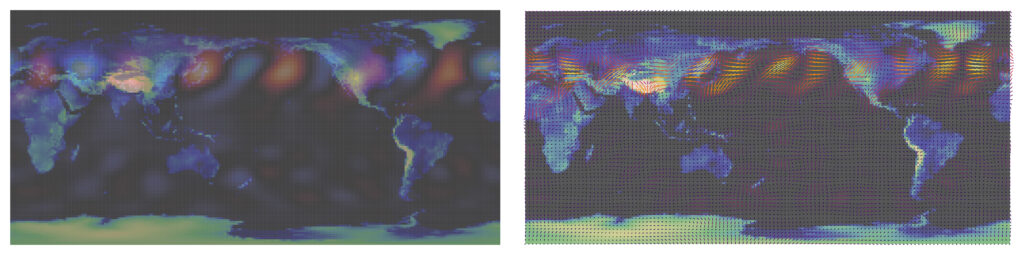Exemplary scalar and vector fields. Vector components of the wind velocities (right) are strongly related, i.e. they form a vector field. Additionally, the wind vector field and the scalar pressure field (left) are related since the gradient of the pressure field causes air movement and subsequently influences the wind components.

Most current methods do not explicitly take into account the relationship between different fields and their internal components, which are often correlated. In this work, we propose to view the time evolution of such correlated fields through the lens of multivector fields. Multivector fields consist of scalar, vector, as well as higher-order components, such as bivectors and trivectors. Their algebraic properties, such as multiplication, addition and other arithmetic operations can be described by Clifford algebras.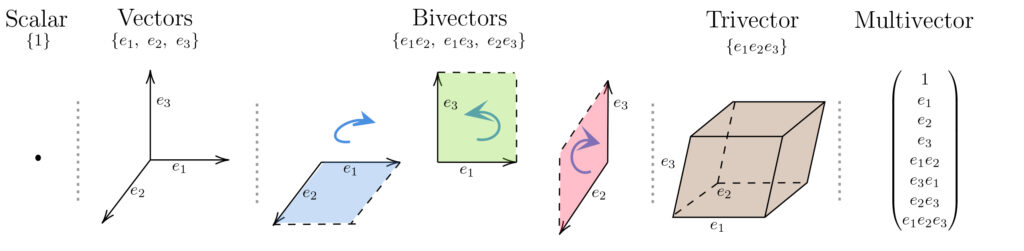Multivector components of 3-dimensional Clifford algebras. In contrast to standard vector algebra, higher order objects such as bivectors and trivectors exist. All spatial primitives can be combined into one multivector.

Clifford algebras allow us to extend operations such as convolutions and Fourier transforms over multivector fields. Consequently, we can build Clifford convolution and Clifford Fourier transform layers. The resulting Clifford neural layers are universally applicable and will find direct use in the areas of fluid dynamics, weather forecasting, and the modeling of physical systems in general.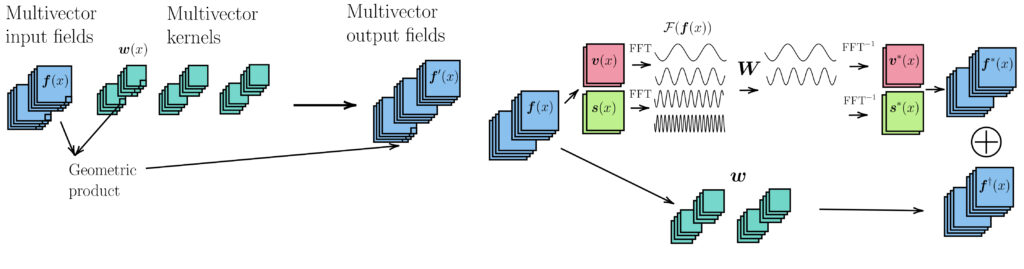Sketch of 2-dimensional Clifford convolution (left) and Clifford Fourier transform layers (right). For Clifford convolution, multivector input fields are convolved with multivector kernels. The Clifford Fourier transforms uses the dual structure of multivectors and performs Fast Fourier Transforms (FFTs) over the dual parts.

We empirically evaluate the benefit of these layers by replacing convolution and Fourier operations in common neural PDE surrogates by their Clifford counterparts on two-dimensional Navier-Stokes and weather modeling tasks, as well as three-dimensional Maxwell equations. Clifford neural layers consistently improve generalization capabilities of the tested neural PDE surrogates. Probably the most notable performance improvement is observed when modeling Maxwell’s equations in 3 dimensions, which describe the evolution of electric and magnetic field over time. We can treat these 6 field components (three field components each) as one multivector field, and thus operate directly on this multivector field via 3-dimensional Clifford convolutions and Clifford Fourier transforms.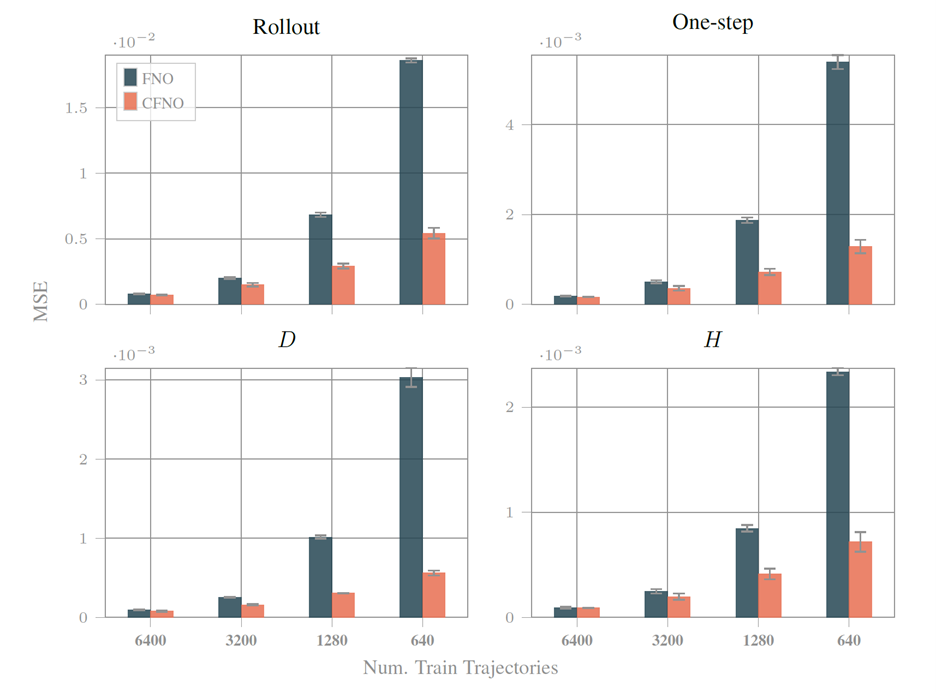Results on the Maxwell equations obtained by Fourier based architectures, i.e., Fourier Neural Operators (FNO) and Clifford Fourier Neural Operators (CFNO). Results are shown for next frame predictions, rollout loss, and displacement field D and magnetization field H. Models are trained on four training sets with increasing number of trajectories.

This work is being undertaken by members of  Microsoft Research AI4Science  & Microsoft Autonomous Systems and Robotics Research,  Johannes Brandstetter,  Rianne van den Berg, Max Welling & Jayesh K. Gupta